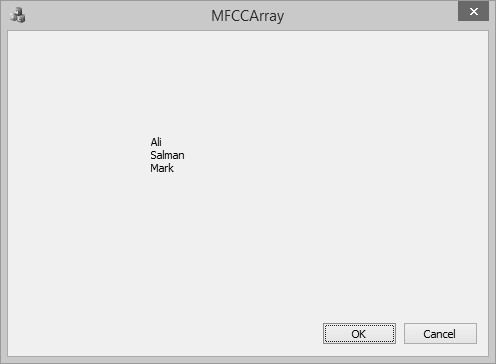# MFC - CArray

CArray is a collection that is best used for data that is to be accessed in a random or non sequential manner. CArray class supports arrays that are like C arrays, but can dynamically shrink and grow as necessary.

• Array indexes always start at position 0.

• You can decide whether to fix the upper bound or enable the array to expand when you add elements past the current bound.

• Memory is allocated contiguously to the upper bound, even if some elements are null.

Following are the different operations on CArray objects −

## Create CArray Object

To create a collection of CArray values or objects, you must first decide the type of values of the collection. You can use one of the existing primitive data types such as int, CString, double etc. as shown below;

```CArray<CString, CString>strArray;
```

To add an item you can use CArray::Add() function. It adds an item at the end of the array. In the OnInitDialog(), CArray object is created and three names are added as shown in the following code.

```CArray<CString, CString>strArray;

```

## Retrieve Items

To retrieve any item, you can use the CArray::GetAt() function. This function takes one integer parameter as an index of the array.

Step 1 − Let us look at a simple example, which will retrieve all the names.

```//Retrive names from CArray
for (int i = 0; i < strArray.GetSize(); i++) {
m_strText.Append(strArray.GetAt(i) + L"\n");
}
```

Step 2 − Here is the complete implementation of CMFCCArrayDlg::OnInitDialog()

```BOOL CMFCCArrayDlg::OnInitDialog() {
CDialogEx::OnInitDialog();

// Set the icon for this dialog. The framework does this automatically
// when the application's main window is not a dialog
SetIcon(m_hIcon, TRUE);               // Set big icon
SetIcon(m_hIcon, FALSE);             // Set small icon

// TODO: Add extra initialization here
CArray<CString, CString>strArray;

//Retrive names from CArray
for (int i = 0; i < strArray.GetSize(); i++) {
m_strText.Append(strArray.GetAt(i) + L"\n");
}

UpdateData(FALSE);
return TRUE; // return TRUE unless you set the focus to a control
}
```

Step 3 − When the above code is compiled and executed, you will see the following output.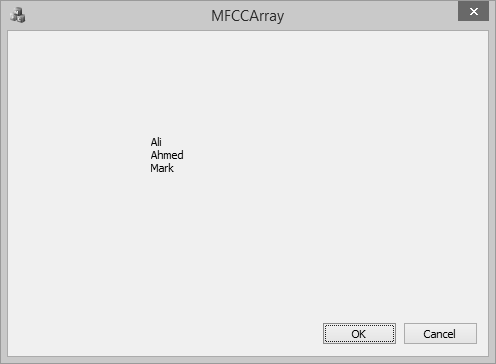## Add Items in the Middle

To add item in the middle of array you can use the CArray::.InsertAt() function. It takes two paramerters — First, the index and Second, the value.

Let us insert a new item at index 1 as shown in the following code.

```BOOL CMFCCArrayDlg::OnInitDialog() {

CDialogEx::OnInitDialog();

// Set the icon for this dialog. The framework does this automatically
// when the application's main window is not a dialog
SetIcon(m_hIcon, TRUE);             // Set big icon
SetIcon(m_hIcon, FALSE);            // Set small icon

// TODO: Add extra initialization here
CArray<CString, CString>strArray;

strArray.InsertAt(1, L"Allan");

//Retrive names from CArray
for (int i = 0; i < strArray.GetSize(); i++) {
m_strText.Append(strArray.GetAt(i) + L"\n");
}

UpdateData(FALSE);
return TRUE; // return TRUE unless you set the focus to a control
}
```

When the above code is compiled and executed, you will see the following output. You can now see the name Allan dded as the second index.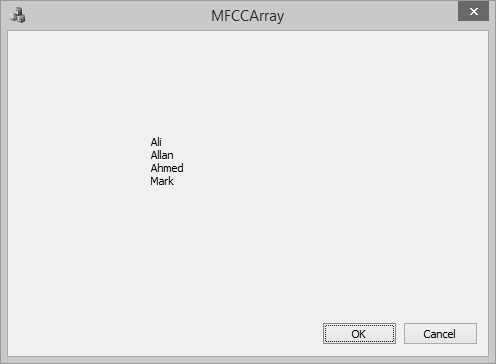## Update Item Value

To update item in the middle of array you can use the CArray::.SetAt() function. It takes two paramerters — First, the index and Second, the value.

Let us update the third element in the array as shown in the following code.

```BOOL CMFCCArrayDlg::OnInitDialog() {
CDialogEx::OnInitDialog();

// Set the icon for this dialog. The framework does this automatically
// when the application's main window is not a dialog
SetIcon(m_hIcon, TRUE);                 // Set big icon
SetIcon(m_hIcon, FALSE);               // Set small icon

// TODO: Add extra initialization here
CArray<CString, CString>strArray;

strArray.InsertAt(1, L"Allan");

strArray.SetAt(2, L"Salman");

//Retrive names from CArray
for (int i = 0; i < strArray.GetSize(); i++) {
m_strText.Append(strArray.GetAt(i) + L"\n");
}

UpdateData(FALSE);
return TRUE; // return TRUE unless you set the focus to a control
}
```

When the above code is compiled and executed, you will see the following output. You can now see that the value of third element is updated.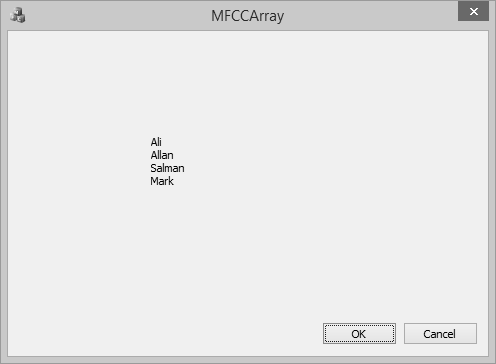## Copy Array

To copy the entire array into another CArray object, you can use CArray::Copy() function.

Step1 − Let us create another array and copy all the elements from first array as shown in the following code.

```BOOL CMFCCArrayDlg::OnInitDialog() {
CDialogEx::OnInitDialog();

// IDM_ABOUTBOX must be in the system command range.
BOOL bNameValid;
ASSERT(bNameValid);
}
}
// Set the icon for this dialog. The framework does this automatically
// when the application's main window is not a dialog
SetIcon(m_hIcon, TRUE);               // Set big icon
SetIcon(m_hIcon, FALSE);              // Set small icon

// TODO: Add extra initialization here
CArray<CString, CString>strArray;

strArray.InsertAt(1, L"Allan");

strArray.SetAt(2, L"Salman");

CArray<CString, CString>strArray2;
strArray2.Copy(strArray);
//Retrive names from CArray
for (int i = 0; i < strArray2.GetSize(); i++) {
m_strText.Append(strArray2.GetAt(i) + L"\n");
}

UpdateData(FALSE);
return TRUE; // return TRUE unless you set the focus to a control
}
```

You can now see that we have retrieved element from the 2nd array and the output is the same because we have used the copy function.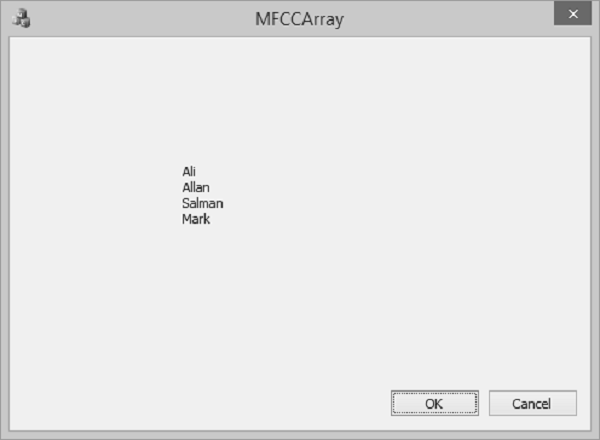## Remove Items

To remove any particular item, you can use CArray::RemoveAt() function. To remove all the element from the list, CArray::RemoveAll() function can be used.

Let us remove the second element from an array.

```BOOL CMFCCArrayDlg::OnInitDialog() {
CDialogEx::OnInitDialog();

SetIcon(m_hIcon, TRUE);             // Set big icon
SetIcon(m_hIcon, FALSE);            // Set small icon

// TODO: Add extra initialization here
CArray<CString, CString>strArray;

strArray.InsertAt(1, L"Allan");

strArray.SetAt(2, L"Salman");

CArray<CString, CString>strArray2;
strArray2.Copy(strArray);

strArray2.RemoveAt(1);

//Retrive names from CArray
for (int i = 0; i < strArray2.GetSize(); i++) {
m_strText.Append(strArray2.GetAt(i) + L"\n");
}

UpdateData(FALSE);
return TRUE; // return TRUE unless you set the focus to a control
}
```

When the above code is compiled and executed, you will see the following output. You can now see that the name Allan is no longer part of the array.